MEMO 2009 pojedinačno problem 3

Kvaliteta:
Avg: 3,0
Težina:
Avg: 6,0
Let$ABCD$ be a convex quadrilateral such that$AB$ and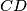$CD$ are not parallel and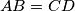$AB=CD$. The midpoints of the diagonals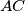$AC$ and$BD$ are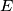$E$ and$F$, respectively. The line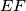$EF$ meets segments$AB$ and$CD$ at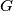$G$ and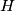$H$, respectively. Show that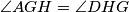$\angle AGH = \angle DHG$.
Izvor: Srednjoeuropska matematička olimpijada 2009, pojedinačno natjecanje, problem 3